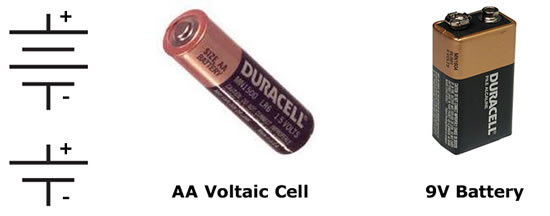# Electrical Circuits

An electrical circuit is a closed loop path through which current can flow. An electrical circuit can be made up of almost any materials (including humans if we're not careful!), but practically speaking, they are typically comprised of electrical devices such as wires, batteries, resistors, and switches. Conventional current will flow through a complete closed-loop path (closed circuit) from high potential to low potential, therefore electrons actually flow in the opposite direction, from low potential to high potential. If there the path isn't a closed loop (open circuit), no charge will flow.Electric circuits, which are three-dimensional constructs, are typically represented in two dimensions using diagrams known as circuit schematics. These schematics are simplified, standardized representations in which common circuit elements are represented with specific symbols, and wires connecting the elements in the circuit are represented by lines. Basic circuit schematic symbols are shown at left.

In order for current to flow through a circuit, you must have a source of potential difference. Typical sources of potential difference are voltaic cells, batteries (which are just two or more cells connected together), and power (voltage) supplies. We often times refer to voltaic cells as batteries in common terminology. In drawing a cell or battery on a circuit schematic, remember that the longer side of the symbol is the positive terminal.Electric circuits must form a complete conducting path in order for current to flow. In the example circuit shown below left, the circuit is incomplete because the switch is open, therefore no current will flow and the lamp will not light. In the circuit below right, however, the switch is closed, creating a closed loop path. Current will flow and the lamp will light up.Note that in the picture at right, conventional current will flow from positive to negative, creating a clockwise current path in the circuit. The actual electrons in the wire, however, are flowing in the opposite direction, or counter-clockwise.

### Energy & Power

Just like mechanical power is the rate at which mechanical energy is expended, electrical power is the rate at which electrical energy is expended. We learned previously that when you do work on something you change its energy, and that electrical work or energy is equal to charge times potential difference. Therefore, we can write our equation for electrical power as:We also know, however, that the amount of charge moving past a point per given unit of time is current, therefore we can continue our derivation as follows:So electrical power expended in a circuit is the electrical current multiplied by potential difference (voltage). Using Ohm's Law, we can expand this even further to provide us with several different methods for calculating electrical power dissipated by a resistor:Of course, conservation of energy still applies, so the energy used in the resistor is converted into heat (in most cases) and light, or it can be used to do work. Let's see if we can't put this knowledge to use in a practical application.Question: A 110-volt toaster oven draws a current of 6 amps on its highest setting as it converted electrical energy into thermal energy. What is the toaster's maximum power rating?

Answer:Question: What is the minimum information needed to determine the power dissipated in a resistor of unknown value?

1. potential difference across the resistor, only
2. current through the resistor, only
3. current and potential difference, only
4. current, potential difference, and time of operation

Answer: (3) current and potential difference, only (P=VI).